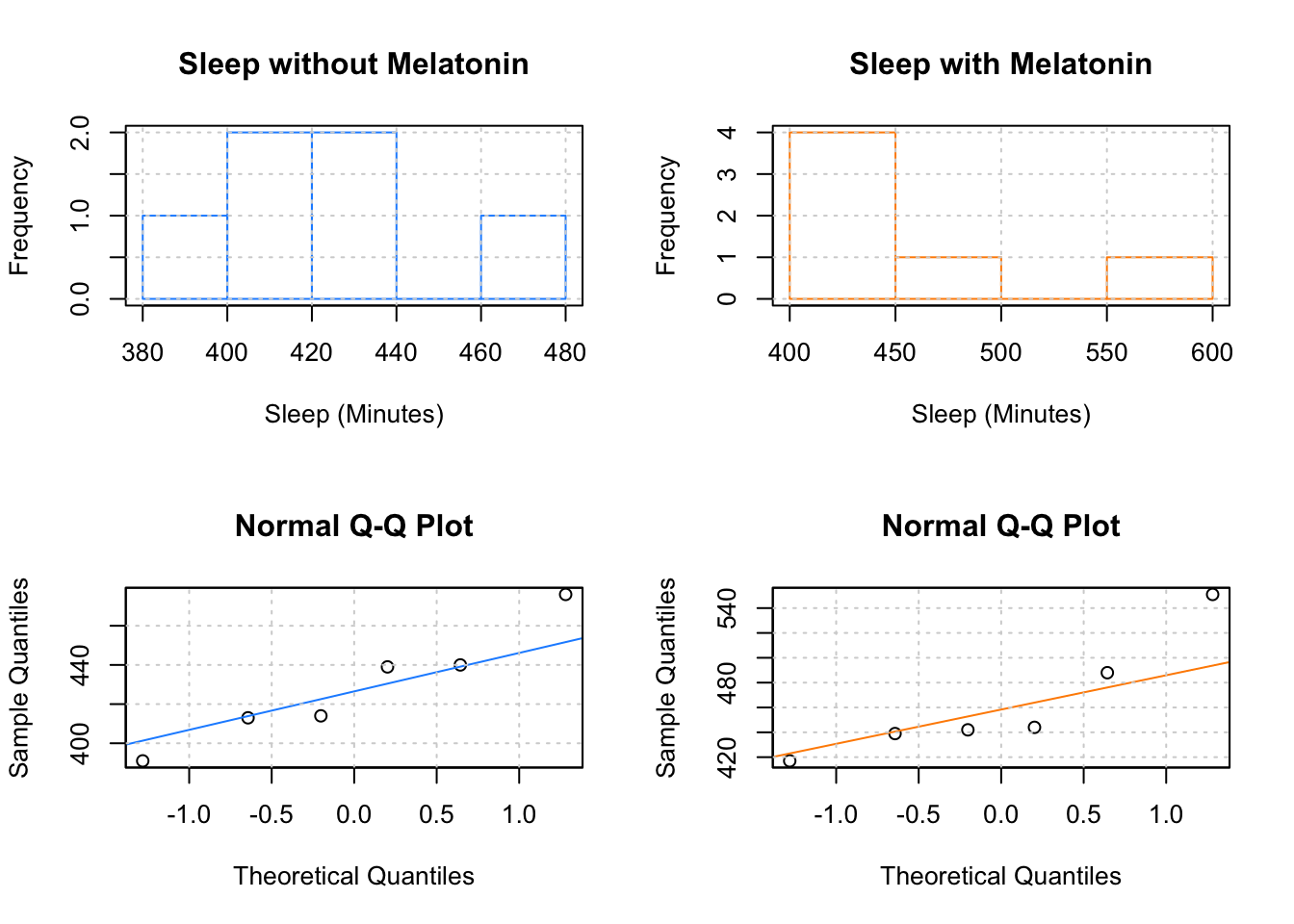# Exercise 1

A track-and-field coach is interested in assessing whether or not a training program is effective. He first records the finishing times for seven 400 meter sprinters in seconds. Much later, after the training program is completed, he records the finishing times of the same seven athletes again. Use the sign test with a significance level of 0.05 to assess whether or not the training regime is effective. That is, test

$H_0\colon \ m_D = m_A - m_B = 0 \quad \text{vs} \quad H_A\colon \ m_D = m_A - m_B \neq 0$

where

• $$m_A$$ is the median finishing time of the 400 meter dash for his athletes after the training program
• $$m_B$$ is the median finishing time of the 400 meter dash for his athletes before the training program

Since it is possible that the training regime makes the runners worse, use a two-sided test.

Pre-Training Finishing Time Post-Training Finishing Time
62 55
72 59
50 48
60 58
62 63
61 59
57 50

For this homework you may use R however you wish. However, since on the exam you may be asked to calculate the p-value “by hand” it is advised to do so for the homework, then check your work using R.

Report:

• The value of the test statistic for the observed data.
• The distribution of the test statistic under the null hypothesis.
• The p-value of the test.
• A decision when $$\alpha = 0.05$$.
• A conclusion in words.

# Exercise 2

Suppose that a sleep researcher is interested in the effect of exogenous melatonin on total sleep time. The researcher records the amount of sleep that six individuals obtain on a particular night. A week later, he repeats the recordings on the same six individuals, this time after administering 10 mg of melatonin one hour before bedtime. The data gathered is:

Sleep, Minutes, Without Melatonin Sleep, Minutes, With Melatonin
440 439
414 444
476 488
439 442
391 417
413 551

Use the sign test with a significance level of 0.05 to assess whether or not melatonin is effective. That is, test

$H_0\colon \ m_D = m_M - m_N = 0 \quad \text{vs} \quad H_A\colon \ m_D = m_M - m_N > 0$

where

• $$m_M$$ is the median sleep time of individuals using melatonin
• $$m_N$$ is the median sleep time of individuals not using melatonin

For this homework you may use R however you wish. However, since on the exam you may be asked to calculate the p-value “by hand” it is advised to do so for the homework, then check your work using R.

Report:

• The value of the test statistic for the observed data.
• The distribution of the test statistic under the null hypothesis.
• The p-value of the test.
• A decision when $$\alpha = 0.10$$.
• A conclusion in words.

# Exercise 3

With the data from Exercise 2, use a paired t-test to test

$H_0\colon \ \mu_D = \mu_M - \mu_N = 0 \quad \text{vs} \quad H_A\colon \ \mu_D = \mu_M - \mu_N > 0$

Report:

• The value of the test statistic for the observed data.
• The distribution of the test statistic under the null hypothesis.
• The p-value of the test. (You will need R to do so. On an exam you may be asked instead to use a critical value.)
• A decision when $$\alpha = 0.10$$.
• A conclusion in words.

# Exercise 4

Even though you could calculate a p-value for both of the previous exercises, does that mean that you should have? In other words, are the two previous tests valid?Using the above plots, and any other information given in the two previous exercises, state a preference for the sign-test or the paired t-test. Justify your answer.

# Exercise 5

An instructor hopes to write an exam with an average score of 85. For this problem, assume that exam scores follow a normal distribution with a variance of 6. That is

$X \sim N(\mu, \sigma^2 = 36)$

This instructor will be administering the exam to 16 students. He decides that if the class average is above an 88, he should not believe that he accomplished his goal of writing an exam with an average of 85, and instead the true average is actually higher. In other words, he is performing the test

$H_0\colon \ \mu = 85 \quad \text{vs} \quad H_A\colon \ \mu > 85$

He will reject the null hypothesis if

$\bar{X} > 88$

(a) Calculate the significance level of this test, $$\alpha$$.

(b) Assuming that the true mean for this exam is 91, calculate the power of this test.